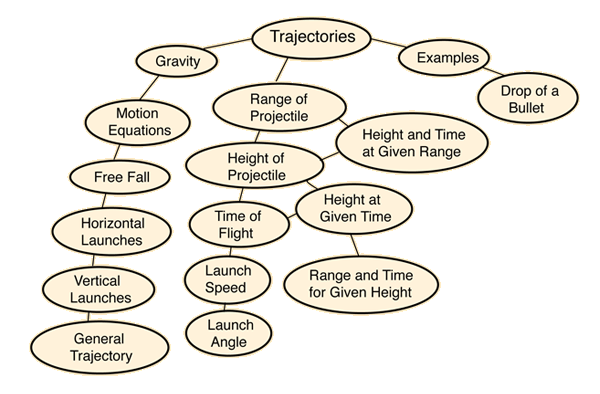Note: This is a large HTML document. Wait until it finishes loading for full functionality.
Index

Motion concepts

 HyperPhysics***** Mechanics R Nave
Go Back# Freefall

In the absence of frictional drag, an object near the surface of the earth will fall with the constant acceleration of gravity g. Position and speed at any time can be calculated from the motion equations.

Illustrated here is the situation where an object is released from rest. Its position and speed can be predicted for any time after that. Since all the quantities are directed downward, that direction is chosen as the positive direction in this case.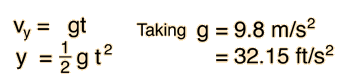At time t = s after being dropped,
the speed is vy = m/s = ft/s ,

The distance from the starting point will be
y = m= ft
Enter data in any box and click outside the box.

Note that you can enter a distance (height) and click outside the box to calculate the freefall time and impact velocity in the absence of air friction. But the calculation assumes that the gravity acceleration is the surface value g = 9.8 m/s2, so if the height is great enough for gravity to have changed significantly the results will be incorrect.

 Free fall with air friction
 Free fall from great height
 Free fall experiment with spark timer
Index

Trajectory concepts

 HyperPhysics***** Mechanics R Nave
Go Back

Peak at m at
t= s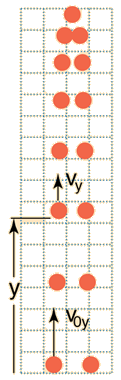# Vertical Trajectory

Vertical motion under the influence of gravity can be described by the basic motion equations. Given the constant acceleration of gravity g, the position and speed at any time can be calculated from the motion equations:You may enter values for launch velocity and time in the boxes below and click outside the box to perform the calculation.

For launch speed v0y = m/s =ft/s
and time t = s ,

The values below are output values; those boxes will not accept input for calculation. The velocity will be
vy = m/s = ft/s
and the height will be y = m = ft
Index

Trajectory concepts

 HyperPhysics***** Mechanics R Nave
Go Back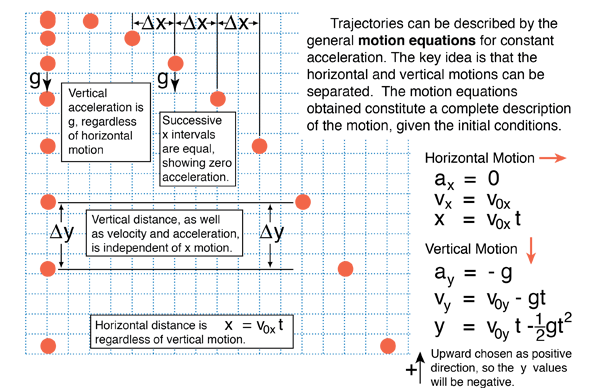Index

Trajectory concepts

Calculation

 HyperPhysics***** Mechanics R Nave
Go Back

# Horizontal Launch

All the parameters of a horizontal launch can be calculated with the motion equations, assuming a downward acceleration of gravity of 9.8 m/s2.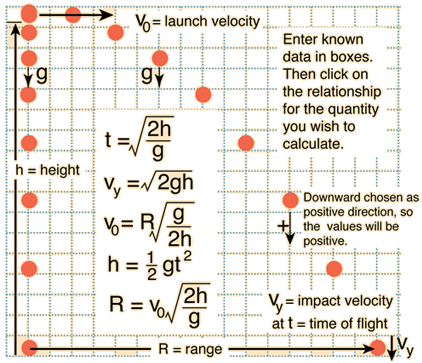Time of flight t = s Vertical impact velocity vy = m/s Launch velocity v0 = m/s Height of launch h = m Horizontal range R = m Calculation is initiated by clicking on the formula in the illustration for the quantity you wish to calculate.
 Include demonstration apparatus
Index

Trajectory concepts

 HyperPhysics***** Mechanics R Nave
Go Back

# General Ballistic Trajectory

The motion of an object under the influence of gravity is determined completely by the acceleration of gravity, its launch speed, and launch angle provided air friction is negligible. The horizontal and vertical motions may be separated and described by the general motion equations for constant acceleration. The initial vector components of the velocity are used in the equations. The diagram shows trajectories with the same launch speed but different launch angles. Note that the 60 and 30 degree trajectories have the same range, as do any pair of launches at complementary angles. The launch at 45 degrees gives the maximum range.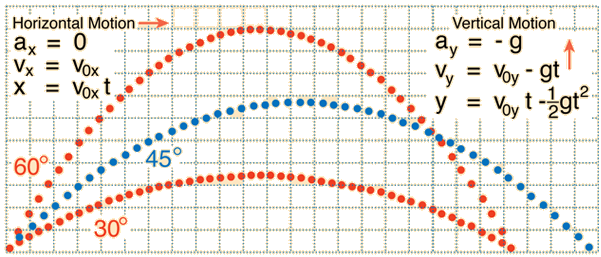Index

Trajectory concepts

Calculation

 HyperPhysics***** Mechanics R Nave
Go BackFor launch velocity v0 = m/s, launch angle θ = degrees: At time t = sec: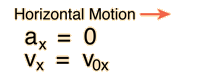Horizontal velocity vx =m/s.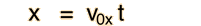Horizontal distance x = m.Vertical velocityvy = m/s.Vertical position y = m.
Index

Trajectory concepts

 HyperPhysics***** Mechanics R Nave
Go Back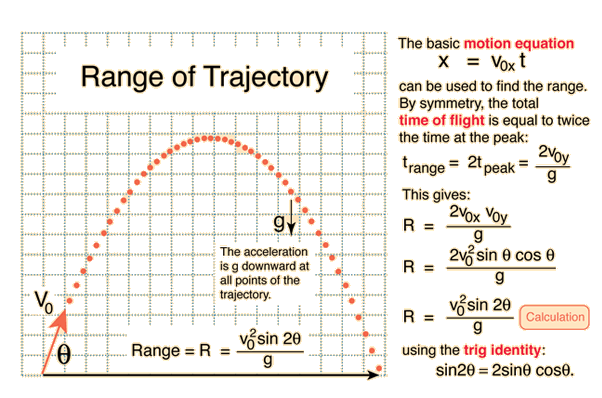Index

Trajectory concepts

 HyperPhysics***** Mechanics R Nave
Go Back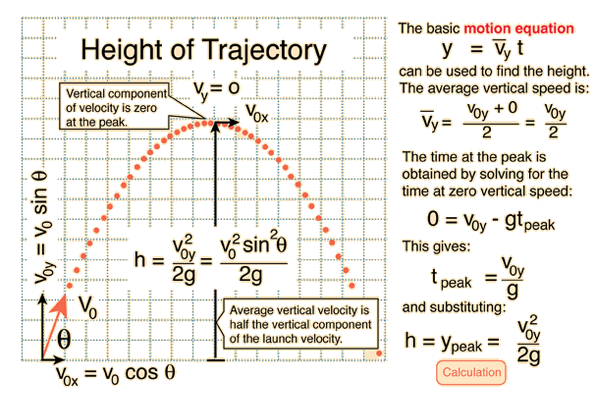Index

Trajectory concepts

 HyperPhysics***** Mechanics R Nave
Go BackIndex

Trajectory concepts

 HyperPhysics***** Mechanics R Nave
Go Back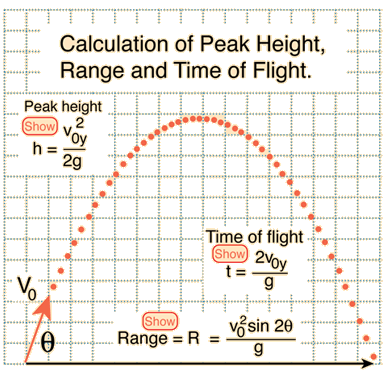For launch velocity v0 = m/s, launch angle θ = degrees, The horizontal range isR = m. The total time of flight is t = s. The peak height ish = m.
Index

Trajectory concepts

 HyperPhysics***** Mechanics R Nave
Go Back

# Will it clear the fence?

The basic motion equations can be solved simultaneously to express y in terms of x.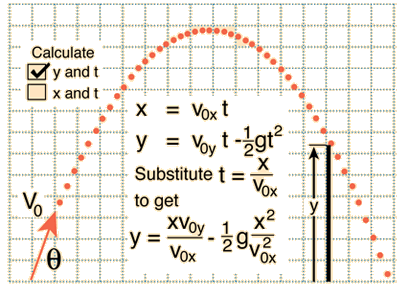For launch velocity v0 = m/s = ft/s, launch angleθ = degrees, and horizontal range x = m = ft, the calculated height is y = m = ft. The time of flight is t = s.
Index

Trajectory concepts

 HyperPhysics***** Mechanics R Nave
Go Back

# Where will it land?

The basic motion equations give the position components x and y in terms of the time. Solving for the horizontal distance in terms of the height y is useful for calculating ranges in situations where the launch point is not at the same level as the landing point.Index

Trajectory concepts

 HyperPhysics***** Mechanics R Nave
Go Back

# Where will it land?

The basic motion equations give the position components x and y in terms of the time. Solving for the horizontal distance in terms of the height y is useful for calculating ranges in situations where the launch point is not at the same level as the landing point.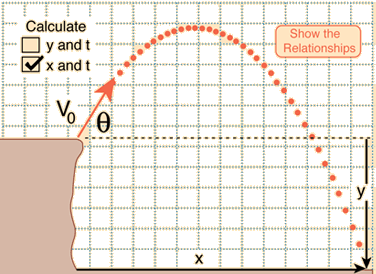Launch velocity v0 = m/s = ft/s, launch angleθ = degrees, and trajectory height y = m = ft,
The two calculated times are
t1 = s and
t2 = s.
The corresponding ranges are
x1 = m =ft
and
x2 = m =ft.
 Note that the value y in the illustration is downward and it is presumed that upward is positive. To reproduce the scenario in the diagram, the input value of y should be negative.
Index

Trajectory concepts

 HyperPhysics***** Mechanics R Nave
Go Back

# Launch Velocity

The launch velocity of a projectile can be calculated from the range if the angle of launch is known. It can also be calculated if the maximum height and range are known, because the angle can be determined.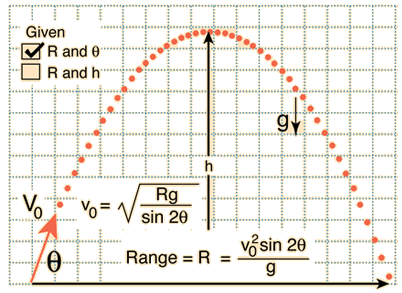From the range relationship, the launch velocity can be calculated. For range R = m = ft, and launch angle θ = degrees, the launch velocity is v0 = m/s = ft/s.
Index

Trajectory concepts

 HyperPhysics***** Mechanics R Nave
Go Back

# Launch Velocity

The launch velocity of a projectile can be calculated from the range if the angle of launch is known. It can also be calculated if the maximum height and range are known, because the angle can be determined.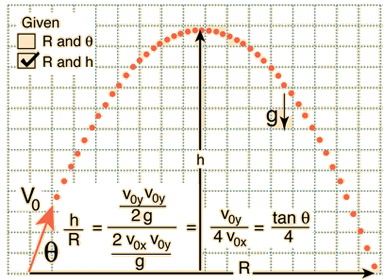For range
R = m = ft,
and peak height
h = m = ft,

the launch velocity is
v0m/s = ft/s.

The required launch angle is
θ = degrees.
Index

Trajectory concepts

 HyperPhysics***** Mechanics R Nave
Go Back

# Angle of Launch

Variation of the launch angle of a projectile will change the range. If the launch velocity is known, the required angle of launch for a desired range can be calculated from the motion equations.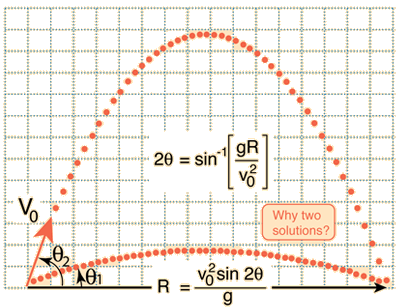From the range relationship, the angle of launch can be determined. For range R = m = ft, and launch velocity v0 = m/s = ft/s. there are two solutions for the launch angle. θ1 = degrees, θ2 = degrees,
Index

Trajectory concepts

 HyperPhysics***** Mechanics R Nave
Go Back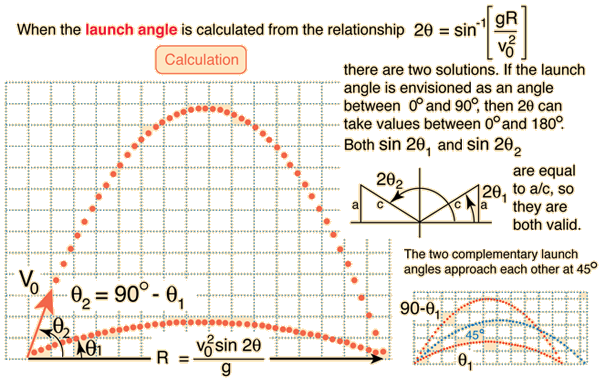Index

Trajectory concepts

 HyperPhysics***** Mechanics R Nave
Go Back